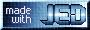# Loving ISIS - Confessions of a Former XSPEC User

Parameters

As discussed on the models page, the model parameters can be edited via the ISIS `edit_par;` command (which will pop up an emacs, vi, jed, etc., window, depending upon the setting of your shell's `EDITOR` environment variable). This is very convenient. Likewise, there is a `set_par()` command that allows parameters to be set on the command line, or in scripts:

```     isis> model("powerlaw(1)");
isis> list_par;
powerlaw(1)
idx  param             tie-to  freeze         value         min         max
1  powerlaw(1).norm       0     0                1           0       1e+10
2  powerlaw(1).PhoIndex   0     0                1          -2           9
isis> set_par(2,1.7,0,1,3);     % set_par(2,1.7,1); would have frozen it
```
I have wrapped a function around this, `newpar()`, which will also take `-1` as indicating a frozen parameter. (Bowing to my XSPEC days...)

The `set_par();` function (or the `newpar();` wrapper) can take either parameter numbers or parameter names (with wild cards!) as inputs:

```     isis> set_par(1,0.1);
isis> set_par("powerlaw(1).norm",0.1);
isis> set_par("pow*norm",0.1);
```
are all equivalent for the above defined model. (Note that the latter function call, however, would set all powerlaw normalization to 0.1, if multiple powerlaws were defined.)

The only exceptions to being able to use a string name for a parameter are when the function or parameter name contains parentheses, `()`, or mathematical operators, e.g., `+`, `/`, `-`, etc. (ISIS will try to parse those as mathematical expressions.) For those cases, one has to use the parameter number.

To save a set of fit parameters to a file `parameter_file.par`, use:

```     isis> save_par("parameter_file.par");
```
To load a set of fit parameters:
```     isis> load_par("parameter_file.par");
```

Manipulating Parameters

Parameters can be tied together, and they can be arbitrary functions of one another. This allows greater flexibility than can be achieved with XSPEC. The ISIS intrinsic command `set_par_fun()` will parse expressions either via `"model_name(id).parameter"`, or via parameter `idx` and expressions using `"_par(idx)"`. (Again, the latter must be used when the function and/or parameter names contains parentheses or mathematical operators.) The former syntax is preferred whenever possible, as parameter ties will then properly update when redefining an existing model definition. The analysis script shows an example.

Here is a toy example that allows one to define a dataset dependent line offset:

```      public define offset ()
{
if (Isis_Active_Dataset == 2)
return 3.0;
else
return 2.5;
}
set_par_fun ("gauss(2).center", "gauss(1).center + offset()");
```
I.e., `Isis_Active_Dataset` is used to define an `offset();` function which has the value 3.0 for dataset 2, but is 2.5 for all other datasets. This function is then used to tie together two gaussian line mid-points.

Next Up: At long last - Fitting!

This page was last updated Oct 7, 2013 by Michael Nowak. To comment on it or the material presented here, send email to mnowak@space.mit.edu.